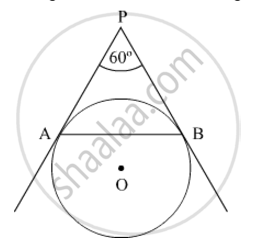# In Fig. 3, AP and BP are tangents to a circle with centre O, such that AP = 5 cm and ∠APB = 60°. Find the length of chord AB. - Mathematics

In Fig. 3, AP and BP are tangents to a circle with centre O, such that AP = 5 cm and ∠APB = 60°. Find the length of chord AB.#### Solution

PA and PB are tangents drawn to the given circle from an external point P.

It is known that the lengths of the tangents drawn from an external point to a circle are equal.

∴ PA = PB

In ∆PAB, sides PA and PB are of the same length.

Hence, ∆PAB is isosceles, with PA = PB and ∠PAB = ∠PBA = x (say).

It is given that

∠APB = 60°

We know that the sum of the angles of a triangle is 180°

In ∆PAB,

∠PAB + ∠PBA + ∠APB = 180°

x + x + 60° = 180°

⇒ 2x = 120°

x = 60°

Thus,

∠PAB = ∠PBA = ∠APB = 60°

Since all angles of ∆PAB are of the same measure, ∆PAB is equilateral, with AP = BP = AB.
It is given that

AP = 5 cm

∴ AB = AP = 5 cm

Thus, the length of the chord AB is 5 cm.

Concept: Areas of Sector and Segment of a Circle
Is there an error in this question or solution?